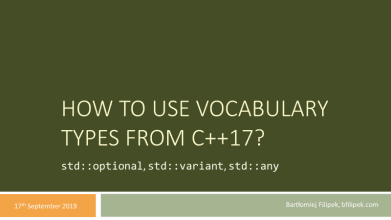# How To Use Vocabulary Types from C++17, Presentation

See my new website at cppstories.comTwo weeks ago, I had a pleasure to give a talk at our local C++ User Group in Cracow. This time I spoke about vocabulary types from C++17: `std::optional`, `std::variant` and `std::any`.

Have a look at the slides and extended comments.

## The Talk

During the presentation, I tried to explain the motivation and some most crucial use cases for the new types that we got in C++17.

For example for `std::variant` we can experiment with the code for computing roots of an equation:

``````// aX^2 + bX + c
? ? ? FindRoots(double a, double b, double c)
{
const auto delta = b * b - 4.0 * a * c;

if (delta > EPSILON)
{
const auto p = sqrt(delta);
const auto x1 = (-b + p) / (2.0 * a);
const auto x2 = (-b - p) / (2.0 * a);
return ? ?
}
else if (delta < -EPSILON)
return ? ? // no roots in real numbers...

return -b / (2.0 * a);
}``````

What type should we return? How to transfer the information that we might have none, one or two roots of the equation?

Some options:

• use `int` as the return type and return number of roots, then take two double pointers or references to output the results.
• return `vector<double>` with the results
• return `tuple<int, double, double>` (integer for the number of results and then two possible values).

Or we can use `std::variant`:

``````// aX^2 + bX + c
using TRoots = std::variant<std::monostate,
double,
std::pair<double, double>>;

TRoots FindRoots(double a, double b, double c)
{
const auto delta = b*b-4.0*a*c;

if (delta > EPSILON)
{
auto p = sqrt(delta);
double x1 = (-b + p)/(2*a);
double x2 = (-b - p)/(2*a);
return std::pair(x1, x2);
}
else if (delta < -EPSILON)
return std::monostate();

return -b/(2*a);
}``````

Now, with `std::variant` we can express all the possible options without any hacks or output parameters.

What do you think about such design?

## Extra Info

If you want to learn more about those types, you can also read the following blog posts:

Plus each of the types has a separate chapter in my book:

If you want to get additional C++ resources, exlusive articles, early access content, private Discord server and weekly curated news, check out my Patreon website: (see all benefits):